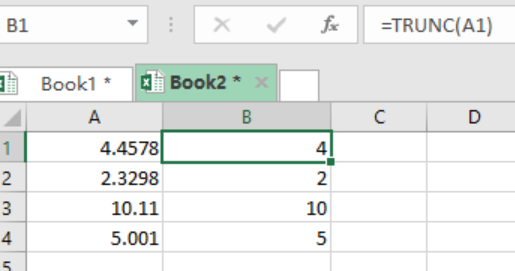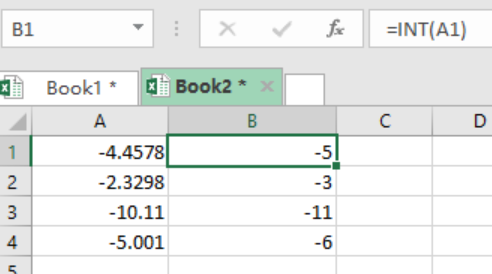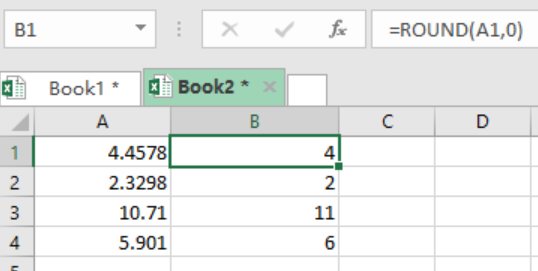Get instant live expert help with Excel or Google Sheets“My Excelchat expert helped me in less than 20 minutes, saving me what would have been 5 hours of work!”

#### Post your problem and you’ll get expert help in seconds.

Your message must be at least 40 characters
Our professional experts are available now. Your privacy is guaranteed.

# Get integer part of a number

We can use the TRUNC function to remove the decimal part of a number and only get the integer portion. This post provides an step by step guide on how we can get the integer of a number.Figure 1: Using TRUNC to get integer portion of a number

## Syntax of the formula

`=TRUNC(number)`

Number refers to the value that we want to find its integer. We can also use a cell reference in the place of number.

## Explanation

The Excel TRUNC function is useful in slicing the decimal part of a number and returning its integer part. In our example above, the formula in B1 is as below;

`=TRUNC(A1)`

When we press Enter, the formula will slice off the decimal part (.4578) and only returns its integer (4).

Copying the formula across the other cells:

• Select the cell with the formula, i.e. B1.
• Point the cursor to the bottom-left corner of the cell, until we see a plus sign.
• Hold the cursor down and drag it across the other cells.

Note, this function does not round off a number, it only removes the decimal portion.

We can supply a second argument to specify the precision of the truncation, though this is optional. When not supplied, the function treats it as zero. This means that the truncation will happen at the decimal point.

## What of INT or ROUND functions?

With default settings, INT works the same way as TRUNC, when it comes to positive numbers. The only difference is that the INT function will round a number down to the next integer, before returning the integer portion of the number.

### Example 2

=INT(number)Figure 2: INT rounding off numbers away from zero

But for negative numbers, INT function deviates from how TRUNC works. The INT function rounds numbers down away from zero, irrespective of the decimal value.

If we want to round a number to the next integer, whether positive or negative, then use the ROUND function. This function looks as below;

`=ROUND(number, 0)`

### Example 3Figure 3: Using the ROUND function

Most of the time, the problem you will need to solve will be more complex than a simple application of a formula or function. If you want to save hours of research and frustration, try our live Excelchat service! Our Excel Experts are available 24/7 to answer any Excel question you may have. We guarantee a connection within 30 seconds and a customized solution within 20 minutes.

### Did this post not answer your question? Get a solution from connecting with the expert.Another blog reader asked this question today on Excelchat:
Solution examplesI have 2 cells containing a % value. The value for both is 9%. I want to conditionally format these cells as duplicate values but excel only highlights them as duplicate values if the decimal point is exactly the same. How can i correct this?
Solved by S. C. in 40 minsI need help with this formula. I need this to include months =INT((M4)/7)&" weeks"&IF(MOD(M4,7)=0,"",", "&INT(MOD(M4,7))&" days") I have the number of Days to Start with
Solved by F. A. in 29 minsI have an IF statement that works. Its pretty complicated with VLOOKUP. But i need to make this an OR statement and i keep getting errors and can't figure out the formula.
Solved by F. S. in 30 minsI WANT TO WRITE A FORMULA THAT SAYS IF THE NUMBER IN COLUMN A MATCHES THE NUMBER IN COLUMN B THE INSERT THE NUMBER FROM COLUMN C INTO COLUMN D
Solved by A. W. in 20 minsExcel formula returns a lot of #N/A results but unsure how to incorporate the IFERROR function to remove them, Can anybody help? =IF(\$A3="","",IF(VLOOKUP(\$A3,'SHAREPOINT LINK]Jobs Log'!\$A:\$Y,17,FALSE)="","",VLOOKUP(\$A3,'SHAREPOINT LINK]Jobs Log'!\$A:\$Y,17,FALSE))) I have removed the link to the sharepoint doc as it gives away the company name so where it says 'SHAREPOINT LINK' just ignore, I don't think anyone needs to know that info. to get the formula to work. Thanks in advance to anyone able to help.
Solved by X. E. in 5 mins

### Leave a Comment## Subscribe to Excelchat.co

Get updates on helpful Excel topics

## Did this post not answer your question? Get a solution from connecting with the expertAnother blog reader asked this question today on Excelchat:

#### Post your problem and you’ll get expert help in seconds.

Your message must be at least 40 characters
Our professional experts are available now. Your privacy is guaranteed.
Trusted by people who work at